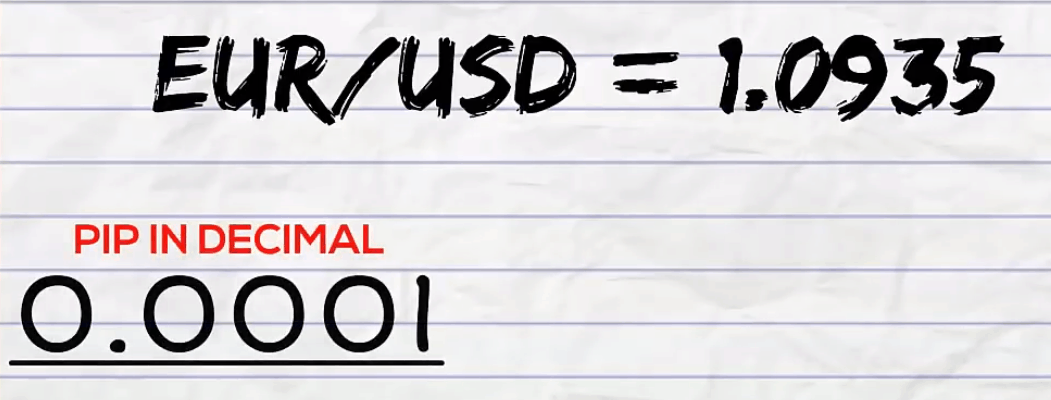# What is a Pip in Forex Trading?

You must’ve heard the term “pip” multiple times. If you’re still not sure what exactly it means, go ahead and read this article. To achieve success in trading, you must have a complete understanding of pips.

Before reading the article and writing your questions in the comments section, I recommend to watch this video. It’s not long but covers the biggest part of questions on the topic.

A pip is a unit of measurement that shows a change in the value of a currency pair. Prices of major currency pairs are displayed with 4 decimal places. A pip is the last decimal place. It amounts to 1/100 of 1% or one basis point.

You might be wondering why traders introduced such a unit in the first place. The thing is that it helps protect investors from severe losses. For example, if a pip amounts to 10 basis points, a 1-pip change can have a major impact on the value of your trade.

On the websites of some Forex brokers, you may come across fractional pips. A fractional pip is equivalent to 1/10 of a pip and is called a “pipette.” In the distance is measured in pipettes, it means that quotes have an extra decimal place. Such quotes are considered more accurate.

Here are the three main factors that determine the monetary value of a pip:

1. currency pair;
3. exchange rate.

Looking at these factors, it’s easy to see that even the slightest fluctuation in the value of a pip can have a dramatic effect on the value of your position.

Let’s take an example. Suppose, you’re trading USD/CAD currency pair. The size of your trade is \$300,000. The trade closed at 1.0568 after a price had covered a 20-pip distance. How do you calculate your profit in USD dollars?

1. First, we need to find out how many Canadian dollars 1 pip is worth. We multiply 1 pip by the size of a trade:
300 000 х 0.0001 = 30 Canadian dollars in 1 pip.
2. Second, we need to find out how many American dollars 1 pip is worth. We divide the CAD amount in 1 pip by the exchange rate (at the time when the trade closed):
30 ÷ 1.0568 = 28.39 US dollars in 1 pip.
3. To calculate our profit, we need to multiply the number of pips by the USD amount in 1 pip:
20 х 28.39 = 567.80 US dollars.## Conclusion

Now that you’ve learned how to determine your profits/losses based on the distance in pips, you might be wondering whether you’ll have to make such calculations for every trade. Luckily, the answer is “no.” Needless to say, it would be tiresome and time-consuming! Forex brokers make these calculations for you automatically. Nonetheless, it doesn’t hurt to know how to calculate your profit all by yourself, without the help of clever algorithms.

SHARE